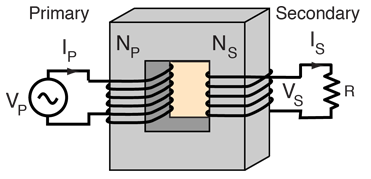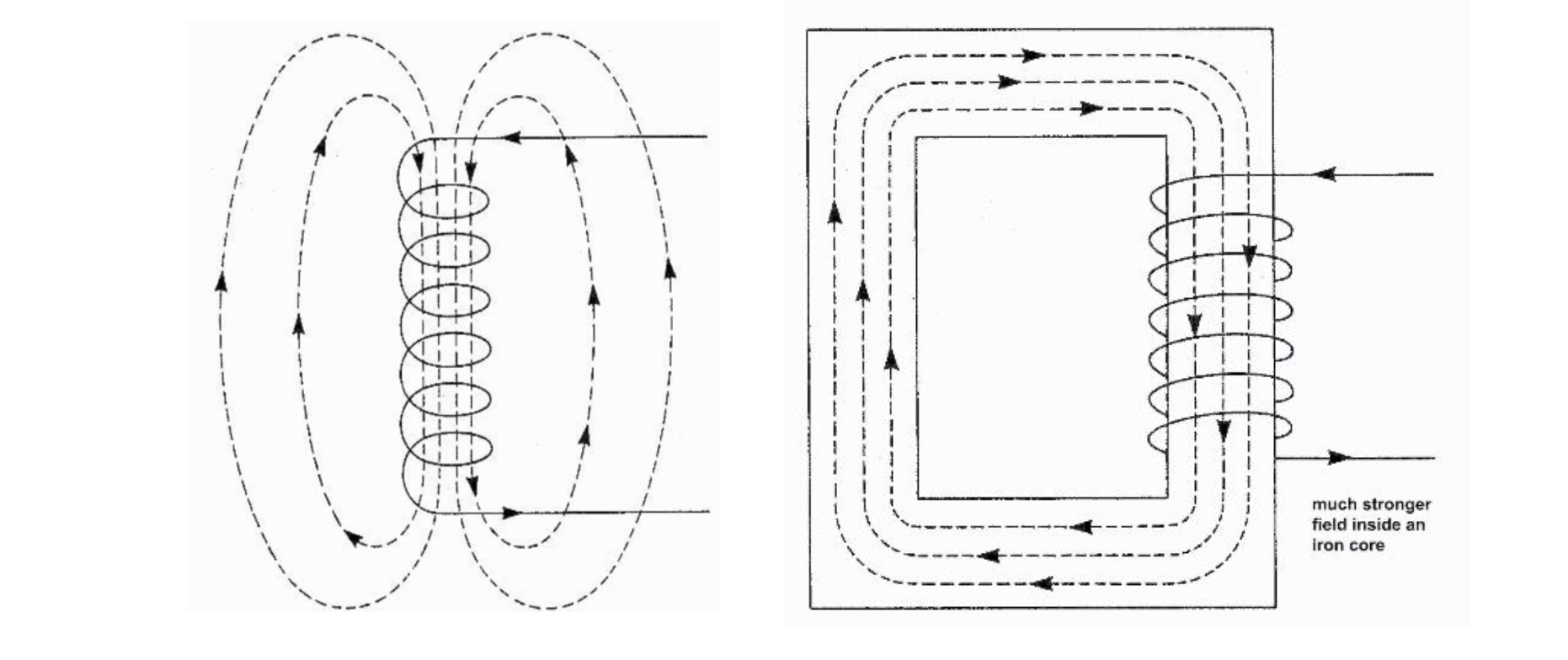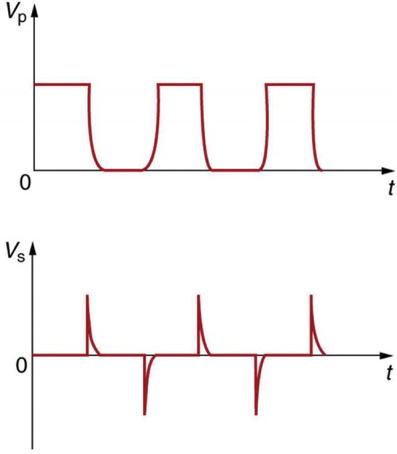# Operation of Ideal Transformers

This is part of the HSC Physics course under the topic Electromagnetic Induction.

### HSC Physics Syllabus

• analyse quantitatively the operation of ideal transformers through the application of: (ACSPH110):
-(V_p)/V_s = (N_p)/(N_s)
-V_pI_p=V_sI_s

### What is a Transformer?

This video will quantitatively analyse the operation of ideal transformers through the application of the following equations: (V_p)/V_s = (N_p)/(N_s) and and V_pI_p=V_sI_s.

### How Do Transformer's Operate?

Transformers operate using Faraday’s Law. An AC supply in the primary coil generates a sinusoidally changing current and thus a magnetic field that constantly changes in orientation and magnitude. The rate of change depends on the AC frequency. The secondary coil experiences the change in magnetic flux and hence a current is induced. Transformers cannot function with a DC current, as it would not cause a change in magnetic flux and Faraday’s law of induction would not apply.A soft iron core is used to magnetically link the primary and secondary coils. The magnetic permeability of iron is much higher than that of air. This means that the magnetic field lines prefer to flow through the iron core instead of air, and are thus directed into the secondary coil.

Iron is also a ferromagnetic material, producing its own magnetic field which aligns with that of the primary coils. As such, the magnetic field is also strengthened.### Quantitative Analysis of Ideal Transformers

The operation of ideal transformers can be quantitatively analysed through the 'transformer equations'. Firstly, we can derive that the ratio of turns of primary and secondary coil dictates the ratio of potential difference (voltage) of the two coils.

$$V_p = - N_p \frac{∆\Phi}{∆t}$$

$$V_s = - N_s \frac{∆\Phi}{∆t}$$

Since the change in magnetic flux experienced by the secondary coil equals the change in flux produced by the primary coil:

$$-\frac{V_p}{N_p}∆t=-\frac{V_s}{N_s}∆t$$

$$\frac{V_p}{N_p}=\frac{V_s}{N_s}$$

We can then derive that the voltage and currents are inversely proportional. Given that the transformer is 'ideal' and has 100% efficiency during this process of electromagnetic induction, then the power input in the primary coils will equal the power output of the secondary coils:

$$P_p = P_s$$

$$V_pI_p = V_sI_s$$

Rearranging gives:

$$\frac{V_p}{V_s} = \frac{I_s}{I_p}$$

The two equations we derived can be combined to give:

$$\frac{N_p}{N_s} = \frac{V_p}{V_s} = \frac{I_s}{I_p}$$

### How Does Lenz's Law Apply in Transformers?

Lenz’s law applies in transformers in that the induced current in the secondary coil is always opposite in direction as the one in primary coil. This causes the two currents to be out-of-phase.Technically, DC supply can induce a current via a non-practical and non-sustainable strategy. Constantly switching on and off a DC supply causes the magnetic field to appear and disappear. This generates a ‘change’ in magnetic flux experienced by the secondary coil, as shown below.﻿